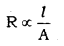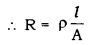# 1. List the factors on which the resistance of a conductor in the shape of a wire depends

1. List the factors on which the resistance of a conductor in the shape of a wire depends.
2. Why are metals good conductors of electricity whereas glass is a bad conductor of electricity? Give reasons.
3. Why are alloys commonly used in electrical heating devices? Give reason.

1.Resistance of conductor depends on following factor:

• Resistance of conductor is directly proportional to length (1) of the conductor.
R ∝ l
• Resistance of conductor is inversely proportional to area of cross section of conductor.• Resistance also depends on a material of conductor §.• Resistance and resistivity also depends on temperature.

2.Metals have more free electrons than glass to carry currents. That’s why glass is bad conductor and metals are good conductors.

3.Alloys are used rather than pure metals in electrical heating devices, since they have low electrical conductivity and also low melting point.